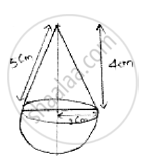Share

# A Toy is in the Form of a Cone Surmounted on a Hemisphere. the Diameter of the Base and the Height of Cone Are 6cm and 4cm. Determine Surface Area of Toy? - CBSE Class 10 - Mathematics

ConceptSurface Area of a Combination of Solids

#### Question

A toy is in the form of a cone surmounted on a hemisphere. The diameter of the base and the height of cone are 6cm and 4cm. determine surface area of toy?

#### Solution

Given height of cone (h) = 4cm

Diameter of cone (d) = 6cm

∴Radius (r) =6/2 =3 cmLet ‘l’ be slant height of cone

l=sqrt(r^2+h^2)

=sqrt(3^2+4^2) =5cm

l = 5cm

∴ Slant height of cone (l) = 5cm.

Curved surface area of cone (S1) = πrl

S1 = π(3)(5) = 47.1cm2

Curved surface area of hemisphere (S2) = 2πr2

S_2=2pi(3)^2=56.52cm^2

∴Total surface area(S) = S1 + S2

= 47.1 + 56.52

= 103.62cm2

∴ Curved surface area of toy = 103.62cm2

Is there an error in this question or solution?

#### Video TutorialsVIEW ALL 

Solution A Toy is in the Form of a Cone Surmounted on a Hemisphere. the Diameter of the Base and the Height of Cone Are 6cm and 4cm. Determine Surface Area of Toy? Concept: Surface Area of a Combination of Solids.
S